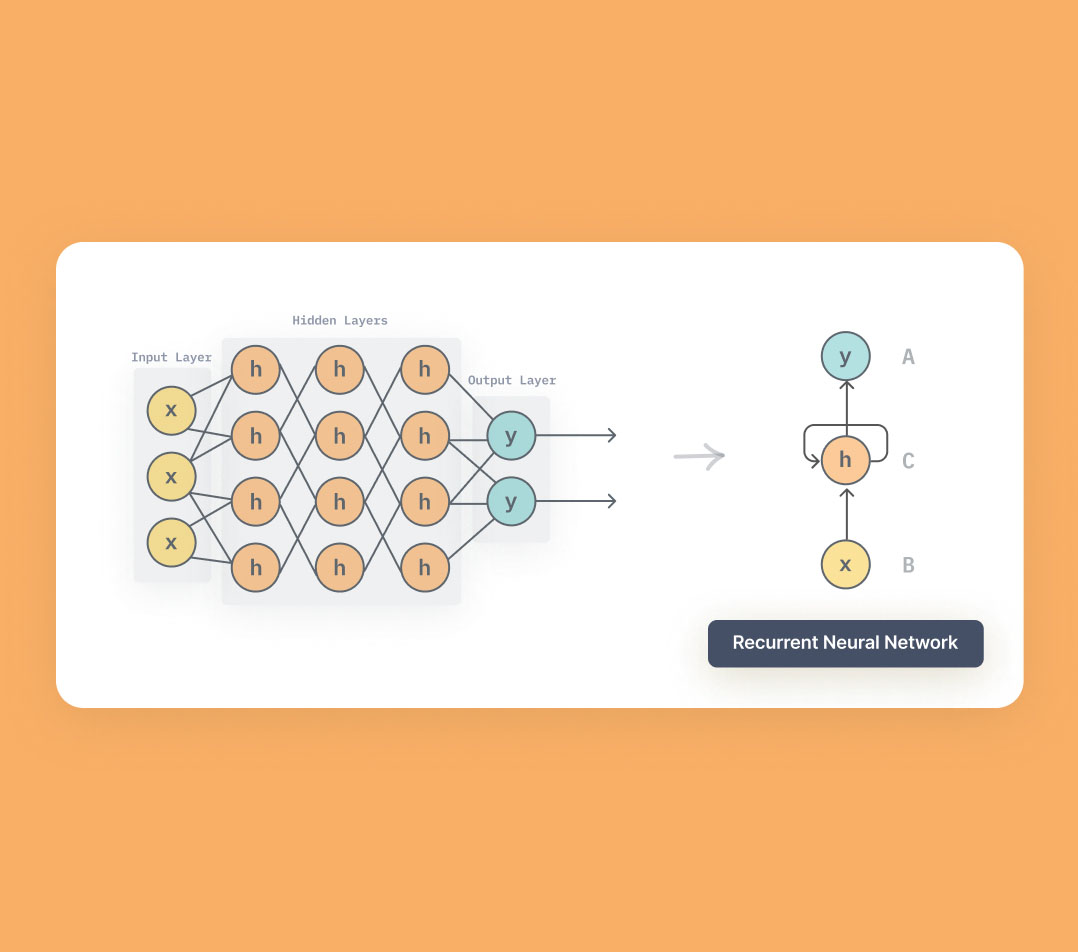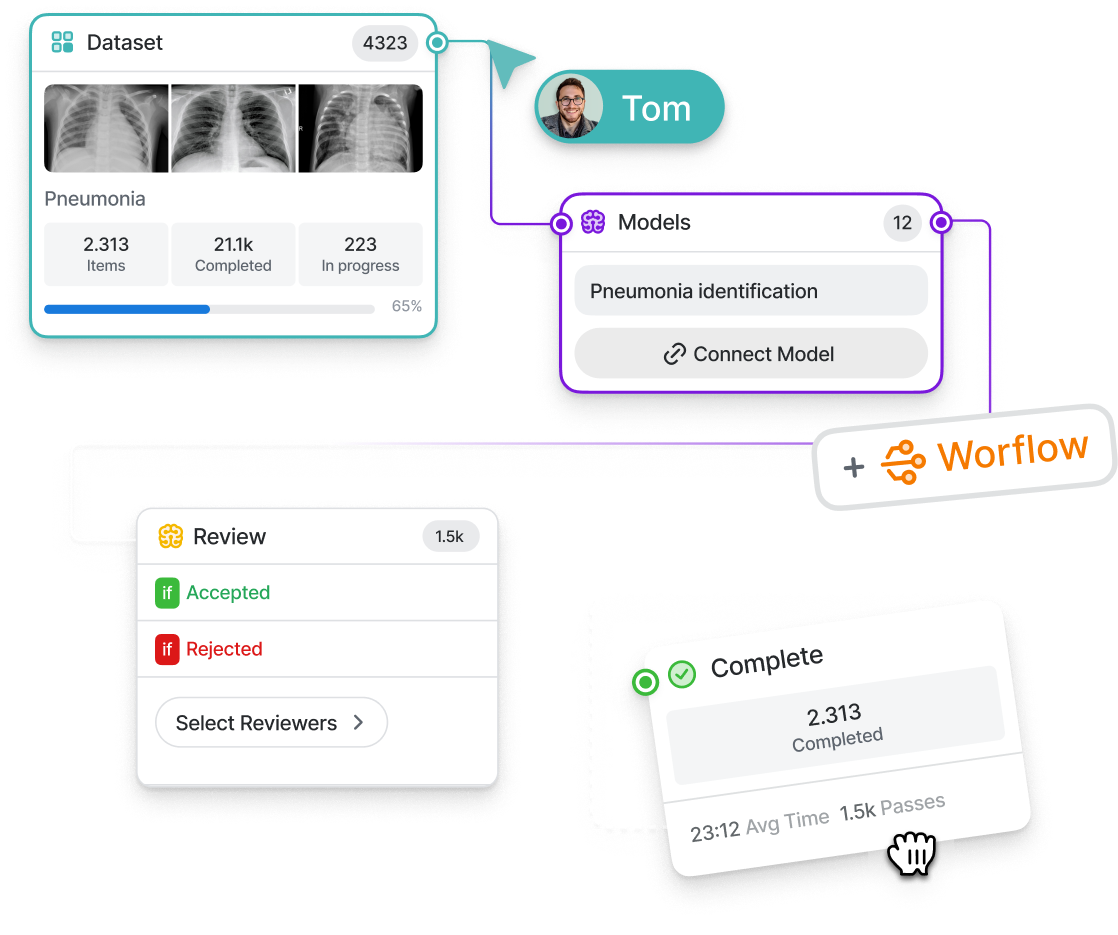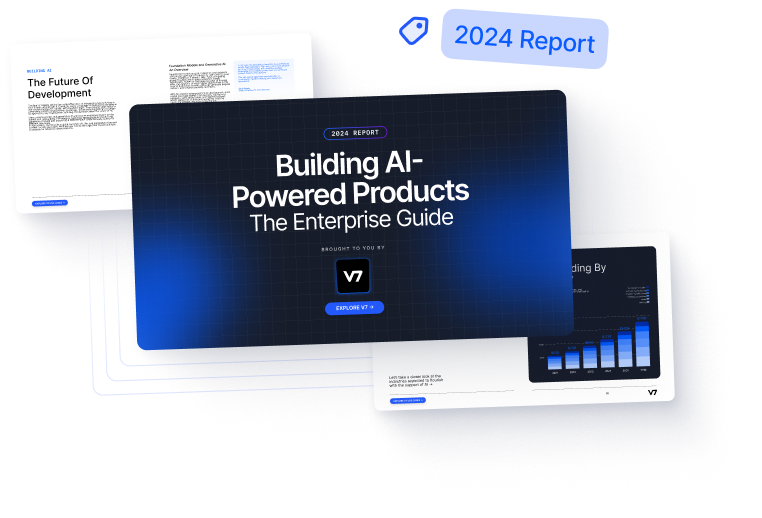# The Complete Guide to Recurrent Neural Networks

Recurrent neural networks (RNNs) are well-suited for processing sequences of data. Explore different types of RNNs and how they work.8
July 29, 2022In order to understand different machine learning algorithms, it is important to first understand the different data types and how they can be processed to train the model. We have seen  Convolutional Neural Networks being used mainly for image/video data. Similarly supervised machine learning algorithms finds application in classification problems where data is structured like in a tabular format.

But, what do you do if patterns in your data change with time and sequential information comes into play? The best bet in such scenarios is to use Recurrent Neural Networks. These have the power to remember what it has learned in the past and apply it in future predictions.

When you surf the internet, the odds are very high that you use applications incorporating Recurrent Neural Networks such as Siri, voice search, and Google Translate. Let’s explore it in more detail.

Here’s what we’ll cover:

Train ML models and solve any computer vision task faster with V7.

Don't start empty-handed. Explore our repository of 500+ open datasets and test-drive V7's tools.

Ready to streamline AI product deployment right away? Check out:

## What are Recurrent Neural Networks?

Recurrent Neural Network is a type of Artificial Neural Network that are good at modeling sequential data. Traditional Deep Neural Networks assume that inputs and outputs are independent of each other, the output of Recurrent Neural Networks depend on the prior elements within the sequence. They have an inherent “memory” as they take information from prior inputs to influence the current input and output. One can think of this as a hidden layer that remembers information through the passage of time.

### Recurrent Neural Networks vs. Feedforward Neural Networks

Feedforward Artificial Neural Networks allow data to flow only in one direction i.e. from input to output. The architecture of this network follows a top-down approach and has no loops i.e., the output of any layer does not affect that same layer. They are mainly used in pattern recognition.

Recurrent Neural Networks have signals traveling in both directions by using feedback loops in the network. Features derived from earlier input are fed back into the network which gives them an ability to memorize. These interactive networks are dynamic due to the ever-changing state until they reach an equilibrium point. These networks are mainly used in sequential autocorrelative data like time series.

### Unfolding Recurrent Neural Networks

As we have already seen, Recurrent Neural Networks are a type of Neural Networks that have an internal memory and function in a way such that the outputs from previous time steps are taken as inputs for the current time step as shown in the below figure.

RNNs are mainly used for predictions of sequential data over many time steps. A simplified way of representing the Recurrent Neural Network is by unfolding/unrolling the RNN over the input sequence. For example, if we feed a sentence as input to the Recurrent Neural Network that has 10 words, the network would be unfolded such that it has 10 neural network layers.

### Advantages and drawbacks of RNNs

• RNN architecture is designed it such a way than it can process inputs of any length. Even with the input size growing larger, the model size does not increase.
• An RNN model is modeled to remember each information throughout the time which is very helpful in any time series predictor.
• The weights of all the dependent hidden layers in between can be shared across the time steps.
• The internal memory of Recurrent Neural Networks is an inherent property that is used for processing the arbitrary series of inputs which is not the case with feedforward neural networks.
• RNNs when combined with traditional Convolutinal Neural Networks gives an effective pixel neighborhood prediction.

• Due to its recurrent nature, the computation becomes slow.
• Training of RNN models can be very difficult and time-consuming as compared to other Artificial Neural Networks.
• It becomes very difficult to process sequences that are very long if the activation functions used are ReLu or tanh as activation functions
• Prone to problems such as exploding and gradient vanishing.
• RNNs cannot be stacked into very deep models
• RNNs are not able to keep track of long-term dependencies

## Types of Recurrent Neural Networks

Traditional Neural networks have independent input and output layers, which make them incompetent when dealing with sequential data. Recurrent Neural Network was introduced to store results of previous outputs in the internal memory. The four commonly used types of Recurrent Neural Networks are:

### One-to-one

The most straightforward type of RNN is One-to-One, which allows a single input and a single output. It has fixed input and output sizes and acts as a standard neural network. The One-to-One application can be found in Image Classification.

### One-to-Many

One-to-Many is a type of RNN that expects multiple outputs on a single input given to the model. The input size is fixed and gives a series of data outputs. Its applications can be found in applications like Music Generation and Image Captioning.

### Many-to-one

Many-to-One RNN converges a sequence of inputs into a single output by a series of hidden layers learning the features. Sentiment Analysis is a common example of this type of Recurrent Neural Network.

### Many-to-many

Many-to-Many is used to generate a sequence of output data from a sequence of input units. It is further divided into ﻿the following two subcategories

• Equal Size: In this case, the input and output layer size is exactly the same.
• Unequal Size: In this case, inputs and outputs have different numbers of units. Its application can be found in Machine Translation.
##### 💡 Pro-tip: Explore V7 hosted 65+ Best compiled datasets for your machine learning projects that are absolutely free.
Build ML workflows. Deploy AI faster.

Plot the best routes for your training data with 8 workflow stages to arrange, connect, and loop any way you need.## Standard RNN’s challenges

Training a RNN or be it any Neural Network is done by defining a loss function that measures the error/deviation between the predicted value and the ground truth. The input features are passed through multiple hidden layers consisting of different/same activation functions and the output is predicted. The total loss function is computed and this marks the forward pass finished. The second part of the training is the backward pass where the various derivatives are calculated. This training becomes all the more complex in Recurrent Neural Networks processing sequential time-sequence data as the model backpropagate the gradients through all the hidden layers and also through time. Hence, in each time step it has to sum up all the previous contributions until the current timestamp.

In some cases the value of the gradients keep on getting larger and becomes infinity exponentially fast causing very large weight updates and gradient descent to diverge making the training process very unstable. This problem is called the exploding gradient.

In some other cases, as the background propagation advances from the output layer to the input layer, the gradient term goes to zero exponentially fast, which which eventually leaves the weights of the initial or lower layers nearly unchange and makes it difficult to learn some long period dependencies.  As a result, the gradient descent never converges to the optimum. This problem is called the vanishing gradient.

## Recurrent Neural Networks Architectures

### Bidirectional recurrent neural networks (BRNN)

A typical RNN relies on past and present events. However, there can be situations where a prediction depends on past, present, and future events.

For example, predicting a word to be included in a sentence might require us to look into the future, i.e., a word in a sentence could depend on a future event. Such linguistic dependencies are customary in several text prediction tasks.

Thus, capturing and analyzing both past and future events is helpful.

To enable straight (past) and reverse traversal of input (future), Bidirectional RNNs or BRNNs are used. A BRNN is a combination of two RNNs - one RNN moves forward, beginning from the start of the data sequence, and the other, moves backward, beginning from the end of the data sequence. The outputs of the two RNNs are usually concatenated at each time step, though there are other options, e.g. summation. The individual network blocks in a BRNN can either be a traditional RNN, GRU, or LSTM depending upon the use-case.

### Gated Recurrent Units (GRU)

There can be scenarios where learning from the immediately preceding data in a sequence is insufficient. Consider a case where you are trying to predict a sentence from another sentence that was introduced a while back in a book or article. In this case, remembering the immediately preceding data and the earlier ones is crucial. A RNN, owing to the parameter sharing mechanism, uses the same weights at every time step. Thus back propagation makes the gradient either explodes or vanishes, and the neural network doesn’t learn much from the data, which is far from the current position.

GRU uses update and reset gate. Basically, these are two vectors which decide what information should be passed to the output. The special thing about them is that they can be trained to keep long-term information without washing it through time or remove information which is irrelevant to the prediction.

• The update gate is responsible for determining the amount of previous information that needs to pass along the next state.
• The reset gate is used from the model to decide how much of the past information is needed to neglect

### Long Short Term Memory (LSTM)

A LSTM is another variant of Recurrent Neural Network that is capable of learning long-term dependencies. Unlike in an RNN, where there’s a simple layer in a network block, an LSTM block does some additional operations. Using input, output, and forget gates, it remembers the crucial information and forgets the unnecessary information that it learns throughout the network.

• Input gate finds which value from input should be used to modify the memory.
• Forget gate learns what details to be discarded from the block.
• Output gate discovers the input and the memory of the block is used to decide the output.

The key difference between GRU and LSTM is that GRU's architecture has two gates that are reset and update while LSTM has three gates that are input, output, forget. GRU is less complex than LSTM because it has less number of gates. Hence, if the dataset is small then GRU is preferred otherwise LSTM for the larger dataset.

## Key Takeaways

• Recurrent Neural Networks, or RNNs, are a specialized class of neural networks used to process sequential data.
• Modeling sequential data requires persisting the data learned from the previous instances. RNN learns and remembers this data so as to formulate a decision, and this is dependent on the previous learning.
• It implements Parameter Sharing by using same weights at every time stamp so as to accommodate varying lengths of the sequential data for which it makes use of feedback loops.
• One main limitation of RNN is that the gradient either explodes or vanishes; The network doesn’t learn much from the data which is far away from the current position.
• RNNs have short term memory problem. To overcome this problem specialized versions of RNN are created like LSTM, GRU.
• Another limitation of RNN is that it processes inputs in a strict temporal order. This means current input has context of previous inputs but not the future.
• Bidirectional RNN (BRNN) duplicates the RNN processing chain so that inputs are processed in both forward and reverse time order.
• RNNs are widely used in the following domains/ applications: Machine Translation, Speech Recognition, Generating Image Descriptions, Video Tagging, Text Summarization etc.Pragati is a software developer at Microsoft, and a deep learning enthusiast. She writes about the fundamental mathematics behind deep neural networks.

“Collecting user feedback and using human-in-the-loop methods for quality control are crucial for improving Al models over time and ensuring their reliability and safety. Capturing data on the inputs, outputs, user actions, and corrections can help filter and refine the dataset for fine-tuning and developing secure ML solutions.”Name
Company
GUIDE
Building AI-Powered Products: The Enterprise Guide

Building AI products? This guide breaks down the A to Z of delivering an AI success story.

Oops! Something went wrong while submitting the form.## Related articles

Gain control of your training data
15,000+ ML engineers can’t be wrongGUIDE
FREE
Building AI-Powered Products: The Enterprise Guide
A Comprehensive Guide

Building AI products? This guide breaks down the A to Z of delivering an AI success story.